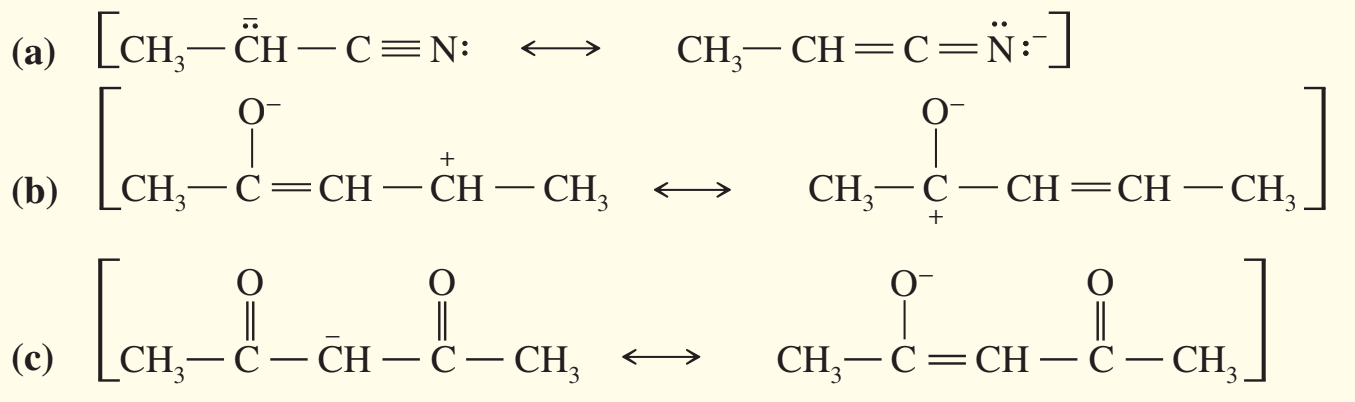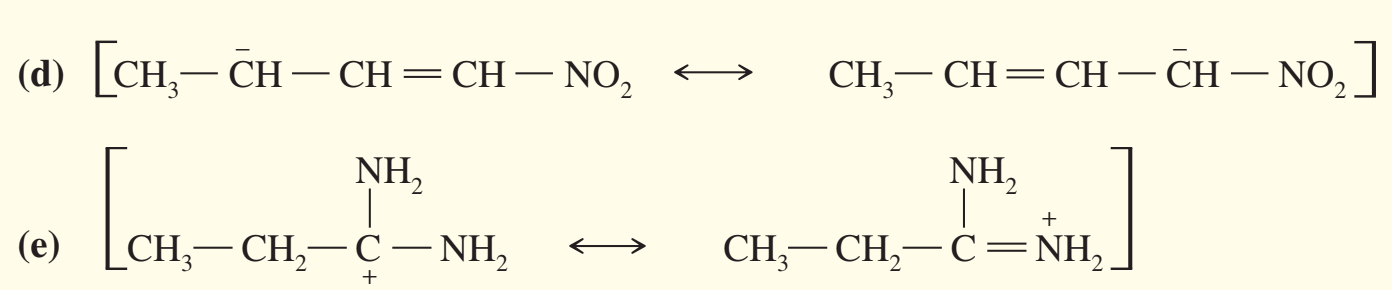×
Get Full Access to Organic Chemistry - 8 Edition - Chapter 1 - Problem 40sp
Get Full Access to Organic Chemistry - 8 Edition - Chapter 1 - Problem 40sp

×

# In the following sets of resonance forms, label the majorISBN: 9780321768414 33

## Solution for problem 40SP Chapter 1

Organic Chemistry | 8th Edition

• Textbook Solutions
• 2901 Step-by-step solutions solved by professors and subject experts
• Get 24/7 help from StudySoup virtual teaching assistantsOrganic Chemistry | 8th Edition

4 5 1 373 Reviews
27
2
Problem 40SP

In the following sets of resonance forms, label the major and minor contributors and state which structures would be of equal energy. Add any missing resonance forms.Step-by-Step Solution:

Solution 40SP:

Here, we are going to find out the major and minor contributor structure of each molecules.

Step 1:

Resonance structures for a molecule would not have same energy.The stable resonance

forms are closer representations of the real molecule than less stable ones.Here, in the first(minor) structures, all the atoms are octet except that central C atom which has nine electrons around it. On the other hand, in the second structures (major), all the carbon atoms are octet except the N atom which has nine electrons around it.

In the in the first(minor) structures, C atom has the negative charge and in the second structure N atom has negative charge on it . Nitrogen  is the more electronegative element, so the second structure is the major contributor.

(b)Here, almost all the atoms are octet in both the structures and negative charge is on the Oxygen atom. Therefore major contributing structure would be that which have minimum charge separation.

Thus, Structure II( Major II) is the major contributing structures.

(c)Here, second (major II)  and third (major III) structures are major. This is because negative charge is on Oxygen atom.

Step 2 of 1

##### ISBN: 9780321768414

This full solution covers the following key subjects: forms, resonance, Major, Energy, equal. This expansive textbook survival guide covers 25 chapters, and 1336 solutions. This textbook survival guide was created for the textbook: Organic Chemistry, edition: 8. Since the solution to 40SP from 1 chapter was answered, more than 716 students have viewed the full step-by-step answer. The full step-by-step solution to problem: 40SP from chapter: 1 was answered by , our top Chemistry solution expert on 05/06/17, 06:41PM. The answer to “?In the following sets of resonance forms, label the major and minor contributors and state which structures would be of equal energy. Add any missing resonance forms.” is broken down into a number of easy to follow steps, and 27 words. Organic Chemistry was written by and is associated to the ISBN: 9780321768414.

## Discover and learn what students are asking

Calculus: Early Transcendental Functions : Hyperbolic Functions
?In Exercises 7-14, verify the identity. $$\cosh ^{2} x=\frac{1+\cosh 2 x}{2}$$

Calculus: Early Transcendental Functions : Differential Equations: Growth and Decay
?In Exercises 1-10, solve the differential equation. $$y^{\prime}=\frac{5 x}{y}$$

Statistics: Informed Decisions Using Data : The Randomized Complete Block Design
?What is blocking? Why might a researcher want to block?

Statistics: Informed Decisions Using Data : Testing the Significance of the Least-Squares Regression Model
?Why is it important to perform graphical as well as analytical analyses when analyzing relations between two quantitative variables?

Unlock Textbook Solution